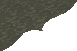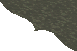Contents Next: Aperiodic Points Up: Classical Results Interpreted Previous: Classical Results Interpreted

## Continued Fractions and Chaos ~~~~~~ Robert M. Corless

The following classical theorem, interpreted in a modern dynamical sense, identifies the fixed and periodic points of the Gauss map.

### Theorem 1

(Galois) The number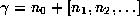has a purely periodic continued fraction, including the first integer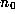, if and only if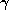is a reduced quadratic irrational.
Corollary: The periodic points of the Gauss map are the reciprocals of the reduced quadratic irrationals.

For a proof of the theorem, see , or . To prove the corollary, we note that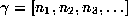is periodic under the Gauss map if and only if its continued fraction is periodic, starting at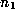, by the shift property mentioned in the previous section.

An example of particular interest is, the golden ratio, which is the positive root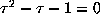. The other root of this quadratic is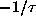which is in the desired interval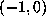. The continued fraction ofis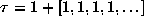, so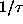has the continued fraction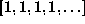, which shows thatis a point of period 1 of the Gauss map. We will return to this example later.

There are general results in the theory of chaotic dynamical systems, with which we could hope to establish the character of the set of periodic points of the Gauss map [24,27,17]. However, these results deal with the characterization of the behaviour of continuous maps of the interval, extended by Block et. al. to maps of the circle , and the Gauss map has a singularity at the origin. Thus the hypotheses of these theorems are not weak enough, so the theorems do not seem to apply. However, the conclusions of these theorems hold, as will be seen by direct methods. This seems to suggest that the theorems may be strengthened somewhat.

We note here that there are infinitely many points of each period. For example,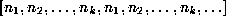has period k, for any choice of integers,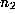,,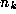. Having points of arbitrary period is one characteristic of a chaotic map . However, we would like to see if the map is sensitive to initial conditions (SIC) in that nearby initial points have orbits that separate at an exponential rate. This again can be established in an elementary fashion by using a classical result.

### Theorem 2

(Lagrange) The numberhas an ultimately periodic continued fraction

if and only ifis a quadratic irrational (is a root of a quadratic with integer coefficients).

Corollary: The Gauss map is SIC.

For a proof of Lagrange's theorem, see . To prove the corollary, we note that every rational initial point is ``attracted'' to the artificial fixed point at 0, while every quadratic irrational is ultimately ``attracted'' to a periodic orbit. Both sets are dense in the interval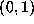. The rate of separation may be checked by considering all points in a small interval I, of width. By the pigeonhole principle, this interval must contain a rational number of the form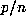(not necessarily in lowest terms), where n is the smallest integer larger than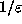. Moreover, we can chooseto be in the interior of the interval, because n is strictly larger than. The number of iterations of the Gauss map required to reach zero from this initial point is, by the speed of the Euclidean algorithm,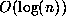and thus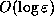. To construct a specific initial point in this interval that does something different under G, first expandinto its finite continued fraction: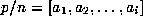. Then for large enough N, the following infinite continued fraction is the continued fraction expansion of a point in I: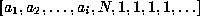. Clearly, the orbit of G starting at this initial point winds up on the fixed point at. Q.E.D.Contents Next: Aperiodic Points Up: Classical Results Interpreted Previous: Classical Results Interpreted